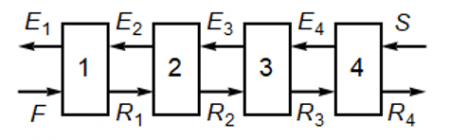LearnChemE

#### Hunter-Nash Method for Liquid-Liquid Extraction: Screencasts

Shows how the mixing point and the operating point in the Hunter-Nash method are determined from mass balances on a two-stage liquid-liquid extraction. The points are located on a ternary phase diagram.

We suggest you list the important points in this screencast as a way to increase retention.

Shows how mass balances and phase equilibrium are used to determine the number of stages in the Hunter-Nash method for liquid-liquid extraction.

We suggest you list the important points in this screencast as a way to increase retention.

##### Important Equations:$\dot F + \dot S = \dot M = \dot E_1 +\dot R_N$

where $$\dot F$$ is the feed flow rate (kg/h)
$$\dot S$$ is the solvent flow rate (kg/h)
$$\dot M$$ is the combined feed plus solvent flow rates (kg/h)
$$\dot E_1$$ is the extract flow rate leaving stage 1
$$\dot R_N$$ is the raffinate flow rate leaving the last stage (the Nth stage). See the above figure.

Mass balances for the case where the solvent contains no solute:

Solute A: $$x^F _A \dot F = x^M _A \dot M$$

Solvent S: $$x^F _S \dot F + \dot S = x^M _S \dot M$$

$x^M _A + x^M _S +x^M _C = 1$

where $$x^i _A, x^i _S$$, and $$x^i _C$$ are the mass fractions of solute, solvent, and carrier, respectively, in the flow stream $$i$$ ($$\dot F, \dot S, \dot M$$).

The mixing point M is located on the ternary phase diagram using the lever rule:

$\frac{\dot S}{\dot F} = \frac{\overline {MF}}{\overline {MS}}$

where $$\overline {MF}$$ is the line segment from the mixing point composition to the feed composition, and $$\overline {MS}$$ is the line segment from the mixing point composition to the solvent composition.

The operating point $$P$$ results from rearranging the overall mass balance ($$\dot F + \dot S = \dot M = \dot E_1 + \dot R_N$$) to yield:

$\dot P = \dot S – \dot R_N = \dot E_1 – \dot F$

This can be arranged to:

$\dot P + \dot R_N = \dot S \,\,and \,\, \dot P + \dot F = \dot E_1$